# QBO Split Training

As with ENSO, we can train QBO on separate intervals and compare the fit on each interval.  The QBO 30 hPa data runs from 1953 to the present.  So we take a pair of intervals — one from 1953-1983 (i.e. lower) and one from 1983-2013 (i.e. higher) — and compare the two.The primary forcing factor is the seasonally aliased nodal or Draconic tide which is shown in the upper left on the figure.  The lower interval fit in BLUE matches extremely well to the higher interval fit in RED, with a correlation coefficient above 0.8.

These two intervals have no inherent correlation other than what can be deduced from the physical behavior generating the time-series.  The other factors are the most common long-period tidal cycles, along with the seasonal factor.  All have good correlations — even the aliased anomalistic tide (lower left), which features a pair of closely separated harmonics, clearly shows strong phase coherence over the two intervals.

That’s what my AGU presentation was about — demonstrating how QBO and ENSO are simply derived from known geophysical forcing mechanisms applied to the fundamental mathematical geophysical fluid dynamics models. Anybody can reproduce the model fit with nothing more than an Excel spreadsheet and a Solver plugin.

Here are the PowerPoint slides from the presentation.

# Short Training Intervals for QBO

[mathjax]An old game show called Name That Tune asked contestants to identify a song by listening to just a few notes.

This post is the QBO equivalent to that. How short an interval can we train on to reproduce the rest of the time series? The answer is not much is required.

# ENSO Proxy Revisited

Although the historical coral proxy measurements are not high resolution (1 year resolution available), they can provide substantiation for models of the modern day instrumental record. This post is a revisit of a previous analysis of the Universal ENSO Proxy (UEP).

The interval from 1881 to 1950 of the ENSO data was used to train the DiffEq ENSO model. This gives a higher correlation coefficient (~0.85) on the test interval (from 1950 to 2014) than the training interval (1881-1950) as shown below:Fig. 1: ENSO model fit over the modern instrumental record

Since ENSO data shows stationarity and coherence over the interval of 70 years, this fit was re-applied to the UEP data over 4 different ranges 1650-1720, 1720-1790, 1790-1860, and 1860-1930. High correlation coefficients were found for each of these intervals (> 0.70) and compared against fits to a red noise model shown below:Fig 2: Proxy fits over various ranges as compared to a red noise model (added for clarification: essentially a synthetic data set to evaluate the ENSO model against). The significance is at least at 0.95 for each proxy interval.

Each of the ENSO fits lies within the 0.95 significance level, and only 1 out of 500 red noise simulations obtained a 0.8 correlation coefficient, which is what the 1720-1790 interval achieved.

Interval CC
1650-1720 0.772
1720-1790 0.807
1790-1860 0.710
1860-1930 0.763

The significance of having each of these intervals at least 0.95 is 1-(1-0.95)^4 if these are all independent. That is a small number less than about (1/20)^4 in likelihood.

The caveat is that the ENSO is also not likely to be coherent over intervals much greater than 70 years, as the shift around 1980 in phase for the model demonstrates.

This substatntiates the finding of Hanson, Brier, Maul  that ENSO and El Nino frequencies may be relatively constant back to the year 1525.

Astudillo et al  also confirmed the ENSO shift after 1980 stating that “the amplitude of the 1982-1983 event is unique”.  Further they concisely describe the ENSO behavior as being highly deterministic by stating:

“This is of crucial importance since if a system is deterministic, the vector field at every period of the state space is uniquely defined by a set of ordinary differential equations.”

## References

 K. Hanson, G. W. Brier, and G. A. Maul, “Evidence of significant nonrandom behavior in the recurrence of strong El Niño between 1525 and 1988,” Geophysical Research Letters, vol. 16, no. 10, pp. 1181–1184, 1989.

 H. Astudillo, R. Abarca-del-Río, and F. Borotto, “Long-term potential nonlinear predictability of El Niño–La Niña events,” Climate Dynamics, pp. 1–11, 2016.

# QBO Disruption?

“One of the earth’s most regular climate cycles is disrupted” issued recently by the UK Met office

Plus all these recent papers:

Newman, P. A., L. Coy, S. Pawson, and L. R. Lait (2016), The anomalous change in the QBO in 2015–2016, Geophys. Res. Lett., 43, 8791–8797, doi:10.1002/2016GL070373.

Dunkerton, T. J. (2016), The Quasi-Biennial Oscillation of 2015-16: Hiccup or Death Spiral?, Geophys. Res. Lett., 43, doi:10.1002/2016GL070921.

An unexpected disruption of the atmospheric quasi-biennial oscillation, Scott M. Osprey, Neal Butchart, Jeff R. Knight, Adam A. Scaife, Kevin Hamilton, James A. Anstey, Verena Schenzinger, Chunxi Zhang, Science, 08 Sep 2016, DOI: 10.1126/science.aah4156

Do strong warm ENSO events control the phase of the stratospheric QBO?, Geophysical Research Letters, Sep 2016, Bo Christiansen, Shuting Yang, Marianne S. Madsen, DOI: 10.1002/2016GL070751

At RealClimate.org someone named Nemesis asked:

What might the implications of a disrupted QBO be? Any idea?

Concerning the QBO disruption that Nemesis mentions above. Does anybody really understand the QBO to begin with? The original theory was developed by the contrarian Richard Lindzen and it really is a limited model if you dig into it. For example, he never could derive the rather obvious period (28 months) of the QBO.

Years ago (circa 1998) the observation was about the “continuing difficulties in obtaining a realistic QBO” yet you continue to find references to “obtaining realistic QBOs”Get a solid theory for QBO in place and only then can you start to reason about anomalies and disruptions that occur. IMO shouldn’t make assertions regarding the source of the latest disruption unless we can agree on the nominal QBO behavior.

# Compact QBO Derivation

I created a QBO page that is a concise derivation of the theory behind the oscillations:

http://contextEarth.com/compact-qbo-derivation/

Four key observations allow this derivation to work

1. Coriolis effect cancels at the equator and use a small angle (in latitude) approximation to capture any differential effect.
2. Identification of wind acceleration and not wind speed as the measure of QBO.
3. Associating a latitudinal displacement with a tidal elevation via a partial derivative expansion to eliminate an otherwise indeterminate parameter.
4. Applying a seasonal aliasing to the lunar tractive forces which ends up perfectly matching the observed QBO period.

These are obscure premises but all are necessary to derive the equations and match to the observations.

This model should have been derived long ago …. that’s what has me stumped. Years ago I spent hours working on transport equations for semiconductor devices so have a good feel for how to handle these kinds of DiffEq’s. You literally had to do this otherwise you would never develop the intuition on how a transistor or some other device works. The QBO for some reason reminds me quite a bit of solving the Hall effect. And of course it has geometric resemblance to the physics behind an electric motor or generator. Maybe I am just using a different lens in solving these kinds of problems.

# ENSO Model Final Stretch (maybe)

[mathjax]I recently posted a bog article called QBO Model Final Stretch. The idea with that post was to give an indication that the physics and analytical math model explaining the behavior of the QBO was in decent shape. I would like to do the same thing with the ENSO model but retain the context of the QBO model.  Understanding the QBO was a boon to making progress with ENSO as it provided an excellent training ground for time-series analysis and also provided some insight into the underlying forcing functions.  In the literature, there is a clear indication that ENSO and QBO are somehow related, but the causality chain remains unclear.

# Geophysical Fluid Dynamics first, and then CFD

A recent perfunctorily-peer-reviewed paper in the Proceedings of the Royal Society

Vallis GK. 2016 “Geophysical fluid dynamics: whence, whither and why?” Proc. R. Soc. A 472: 20160140. http://dx.doi.org/10.1098/rspa.2016.0140 and PDF

explains the distinction between analytical physics models of the climate and purely numerical models — i.e. the field known as computational fluid dynamics (CFD). The lack of an intense review cycle makes for a very readable paper, with a refreshing conversational writing style that the editors apparently allowed. The gist of the piece is that the formulation of analytically-based Geophysical Fluid Dynamics (GFD) models of the atmosphere & ocean are essential to making sure that the CFD’s are on the right track.

# QBO Model Final Stretch

One caveat in this derivation is that the QBO may actually be the $$v(t)$$ term – the horizontal longitudinal velocity of the fluid, the wind in other words – which can be derived from the above by applying the solution to Laplace’s third tidal equation in simplified form above.

Following up on this gap, I extended the derivation to formally evaluate the velocity.

So from:$zeta(t) = sin( sqrt{A} sum_{i=1}^{i=N} k_i sin(omega_i t) + theta_0 )$

and the third simplified Laplace equation$frac {partial v}{partial t} =-frac {1}{a} frac {partial }{partial varphi } (gzeta +U)$

we can derive$frac {partial v}{partial t} = cos( sqrt{A} sum_{i=1}^{i=N} k_i sin(omega_i t) + theta_0 )$

So the acceleration of wind, not the velocity, is what obeys the Sturm-Liouville equation. A derivative preserves the periods of the Fourier components, but not the amplitudes, so what we see is a differently shaped envelope for QBO —  i.e. one that is more spiky due to the time derivative applied.

A single lunar Draconic tidal term of $$lambda_d=27.212$$ days multiplied by a yearly modulation sharply peaked at a specific season is enough to capture the QBO acceleration time-series:$cos^N(2 pi t) cos(frac{2pi}{lambda_d} t)$

The seasonal sharpness is controlled by the power N. This can be approximated as a Fourier series with the same periods found previously: 2.37 years, 0.7 years, 0.41 years, etc.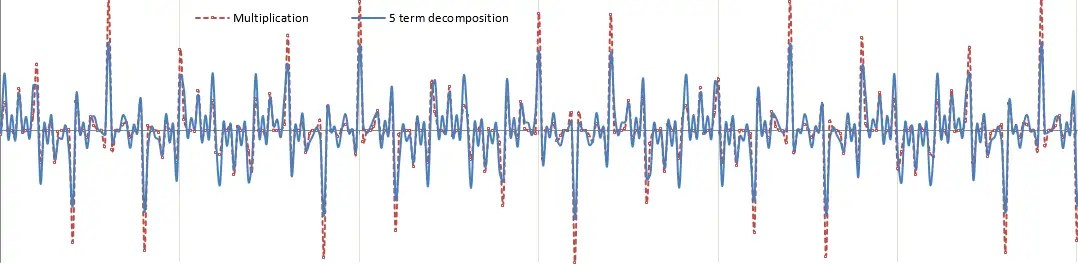Fig. 1: The sesonally modulated lunar cycle can be approximated by a sum of Fourier components

This gives the following fit to the QBO acceleration with a correlation coefficient of 0.35 (good, considering the data waveform has no filtering applied and very little optimization went into the fit):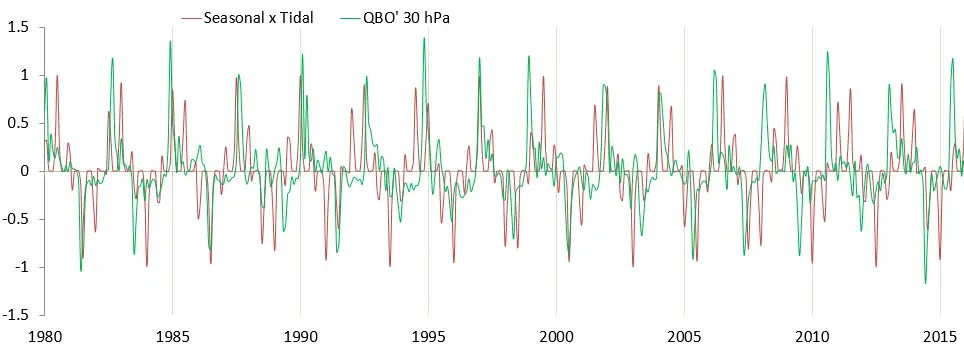Fig 2:  Modulation of Draconic lunar with seasonal period captures the acceleration of the QBO wind.

The excellent model fit makes sense as the acceleration of wind is simply a F=ma response to a gravitational forcing.

The bottom-line is that this acceleration version of the QBO is much more spiky than the more smoothly oscillating conventional wind speed description of QBO. Yet this spikiness is more closely representative of the seasonally modulated lunar forcing. So what was a caveat in the previous post is now a fundamental revelation which provides an excellent modeling path going forward.

Furthermore, a simple time integration of the acceleration model will recover the wind speed version of the QBO. And fortunately this will retain the same Fourier components that we used earlier to model the QBO, so a self-consistency with the prior model of the QBO is retained.

That’s how a solution should ideally converge — unless one has all the pieces simultaneously, incremental changes to an initial model will provide the process to a solution.

Incidentally, I submitted this research to the AGU meeting for this fall and will find out if the abstract gets accepted in October.

8/28/2016: Additional information pulled from an Azimuth Project forum comment

Deriving the QBO from Laplace’s tidal equations, it becomes apparent that the acceleration of wind and not the wind speed is the fundamental measure to characterize. With that premise, things seem to fall into place much more naturally.

First, multiply the nodal/draconic frequency by a sharply peaked yearly modulation and we get the following as a fit for QBO acceleration: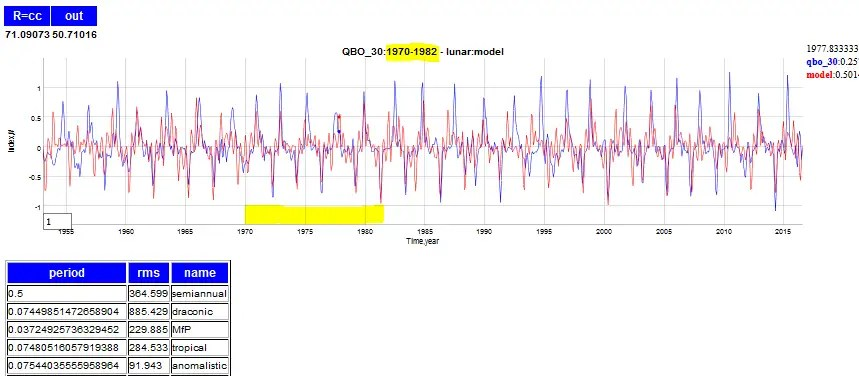The fitting region is 1970 to 1982, and the rest is extrapolated. The correlation coefficient is not extremely high but note the amount of fine detail that gets exposed by the model.

To get back the wind speed QBO, the curves are integratedAgain the model fitting is only conducted on the interval from 1970 to 1982. Outside of that interval the model doesn’t track every peak but enough of the fine detail is captured that it’s obvious that the model has predictive power.

The supporting experiment involves running a symbolic regression machine learning trial on this same interval.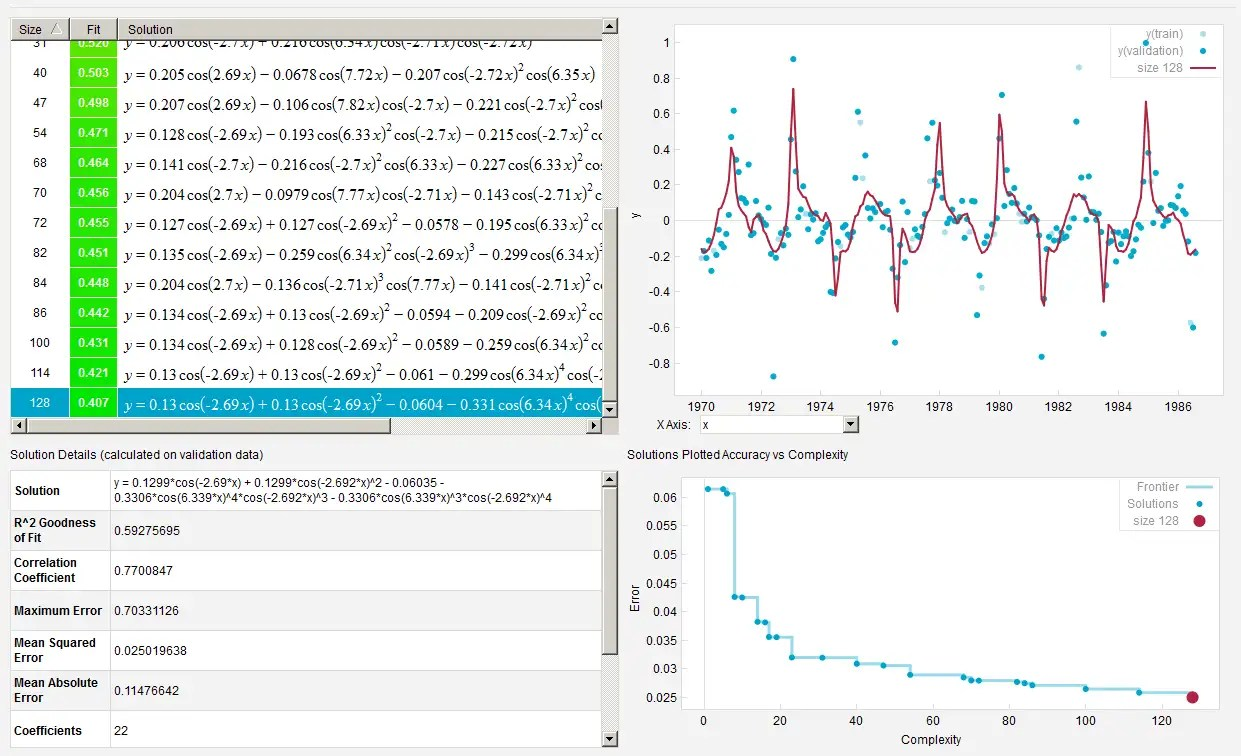This image has been resized to fit in the page. Click to enlarge.

Amazingly, the ML finds all the same harmonics caused by multiplying the sharply peaked yearly signal (freq ~ 2π ) with the Draconic tide (aliased ~2.7 year). No other periods are detected.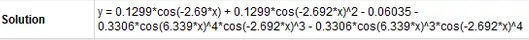# LOD Revisited for CSALT

One of the most questioned aspects of the CSALT model of global temperature is the LOD to Temperature factor. This creates a multi-decadal variation in temperature useful for optimizing a multiple-linear regression AGW model dependent on CO2 and other factors.

Lunisolar tides impact variations in Length-of-Day (LOD). So does ENSO and QBO. There is a recursive aspect to these relationships as well, since both LOD and ENSO have the same Chandler wobble match in apparent forcing periodicity. This is what I believe generates a 6-year signal that gets identified routinely in the LOD time-series, such as the latest finding in ref  below.From ref , the 6-year signal in LOD series. Counting cycles this is close to an average 6.25 year period, close to the 6.4 year Chandler wobble angular momentum variation.

Its a mystery why the LOD may be considered deterministic/periodic based on how well the lunisolar tides resolve the features, but ENSO is only considered quasi-periodic or nearly chaotic (the latter according to Tsonis), even though they likely arise from common mechanisms. Above all, these phenomena all have that curious tie-in to the seasonally aliased Draconic-monthly lunar cycle.

Now we can add this paper by Marcus  to the mix. This is a very detailed look at the correlation between long-range LOD variations (longer than the 6-year variation of Ref ) and global surface temperature. His application of a 5-year running mean is essentially similar to a 5-year lag that works as a best fit in the CSALT model. Marcus stops short of assigning a source cause for the LOD-to-Temperature correlation, but the general idea is that angular momentum variations are the forcing terms that slosh the sources of heat to the surface — “via core-induced rotational and/or related global-scale processes”.  (I also have to note that Marcus is an independent researcher, who at one time had an affiliation with NASA JPL.)From Marcus , correlation of LOD against various temperature indices.

All these observations of LOD, ENSO, QBO, Chandler Wobble, Flood Return periods have a strong sense of self-consistency (IMO ultimately tied to lunisolar forcing), but the problem is that the discussions are scattered among different research groups. And even on this blog, the discussions reside in scattered postings (and over at Azimuth Project, also see another lunar connection).  Eventually I will write a longer manuscript to tie it all together much like I did with The Oil Conundrum and my old fossil-fuel depletion blog.

## References

 Duan, Pengshuo, Genyou Liu, Lintao Liu, Xiaogang Hu, Xiaoguang Hao, Yong Huang, Zhimin Zhang, and Binbin Wang. 2015. “Recovery of the 6-Year Signal in Length of Day and Its Long-Term Decreasing Trend.” Earth, Planets and Space 67 (1): 1.

 Marcus, Steven L. 2015. “Does an Intrinsic Source Generate a Shared Low-Frequency Signature in Earth’s Climate and Rotation Rate?” Earth Interactions 20 (4): 1–14. doi:10.1175/EI-D-15-0014.1.

# Alternate simplification of QBO from Laplace’s Tidal Equations

[mathjax]Here is an alternate derivation of reducing Laplace’s tidal equations along the equator. Recall that the behavior of QBO (and ENSO) is isolated to the equator.

For a fluid sheet of average thickness $$D$$, the vertical tidal elevation $$zeta$$, as well as the horizontal velocity components $$u$$ and $$v$$ (in the latitude $$varphi$$ and longitude $$lambda$$ directions).

This is the set of Laplace’s tidal equations (wikipedia). Along the equator, for $$varphi$$ at zero we can reduce this.{begin{aligned}{frac {partial zeta }{partial t}}+{frac {1}{acos(varphi )}}left[{frac {partial }{partial lambda }}(uD)+{frac {partial }{partial varphi }}left(vDcos(varphi )right)right]=0,\{frac {partial u}{partial t}}-vleft(2Omega sin(varphi )right)+{frac {1}{acos(varphi )}}{frac {partial }{partial lambda }}left(gzeta +Uright)=0,\{frac {partial v}{partial t}}+uleft(2Omega sin(varphi )right)+{frac {1}{a}}{frac {partial }{partial varphi }}left(gzeta +Uright)=0,end{aligned}}

where $$Omega$$ is the angular frequency of the planet’s rotation, $$g$$ is the planet’s gravitational acceleration at the mean ocean surface, $$a$$ is the planetary radius, and $$U$$ is the external gravitational tidal-forcing potential.

The main candidates for removal due to the small-angle approximation along the equator are the second terms in the second and third equation. The plan is to then substitute the isolated $$u$$ and $$v$$ terms into the first equation, after taking another derivative of that equation with respect to $$t$$.{begin{aligned}{frac {partial zeta }{partial t}}+{frac {1}{a}}left[{frac {partial }{partial lambda }}(uD)+{frac {partial }{partial varphi }}left(vDright)right]=0,\{frac {partial u}{partial t}}+{frac {1}{a}}{frac {partial }{partial lambda }}left(gzeta +Uright)=0,\{frac {partial v}{partial t}}+{frac {1}{a}}{frac {partial }{partial varphi }}left(gzeta +Uright)=0,end{aligned}}

Taking another derivative of the first equation:{begin{aligned}{afrac {partial^2 zeta }{partial t^2}}&+ frac {partial }{partial t} left[{frac {partial }{partial lambda }}(uD)+{frac {partial }{partial varphi }}left(vDright)right]=0,end{aligned}}

Next, on the bracketed pair we invert the order of derivatives (and pull out $$D$$, which may not be right for ENSO where the thermocline depth varies!){begin{aligned}{afrac {partial^2 zeta }{partial t^2}}&+ D left[{frac {partial }{partial lambda }}( frac {partial u }{partial t} )+{frac {partial }{partial varphi }}(frac {partial v }{partial t} )right]=0,end{aligned}}

Notice now that the bracketed terms can be replaced by the 2nd and 3rd of Laplace’s equations{begin{aligned}{frac {partial u}{partial t}}&=-{frac {1}{a}}{frac {partial }{partial lambda }}left(gzeta +Uright)end{aligned}}{begin{aligned}{frac {partial v}{partial t}}&=-{frac {1}{a}}{frac {partial }{partial varphi }}left(gzeta +Uright)end{aligned}}

as{begin{aligned}{a^2frac {partial^2 zeta }{partial t^2}}&- D left[{frac {partial }{partial lambda }}( {{frac {partial }{partial lambda }}left(gzeta +Uright)} )+{frac {partial }{partial varphi }}({{frac {partial }{partial varphi }}left(gzeta +Uright)})right]=0end{aligned}}

The $$lambda$$ terms are in longitude so that we can use a separation of variables approach and create a spatial standing wave for QBO, $$SW(s)$$ where $$s$$ is a wavenumber.{begin{aligned}{frac {partial^2 zeta }{partial t^2}}&- D left[( SW(s) zeta )+{frac {partial }{partial varphi }}({{frac {partial }{partial varphi }}left(gzeta +Uright)})right]=0end{aligned}}

The next bit is the connection between a change in latitudinal forcing with a temporal change:begin{aligned} {frac {partial zeta }{partial varphi } = frac {partial zeta }{partial t} frac {partial t }{partial varphi } } end{aligned}

so ifbegin{aligned} frac {partial varphi }{partial t } = sum_{i=1}^{i=N} k_i omega_i cos(omega_i t) end{aligned}

to describe the external gravitational forcing terms, then the solution is the following:begin{aligned} zeta(t) = sin( sqrt{A} sum_{i=1}^{i=N} k_i sin(omega_i t) ) end{aligned}

where $$A$$ is an aggregate of the constants of the differential equation.

So we can eventually get to a fit that looks like the actual QBO, for a fit from 1954 to 2015 for the 30 hPa data.Besides the excursion-limiting behavior imposed by a $$sin(sin(t))$$ modulation, this formulation can also show amplitude folding at the positive and negative extremes. In other words, if the amplitude is too large, the outer $$sin$$ modulation starts to shrink the excursion, instead of just limiting it. Note around the 2016 time frame, the negative peak starts folding inward.

One caveat in this derivation is that the QBO may actually be the $$v(t)$$ term – the horizontal longitudinal velocity of the fluid, the wind in other words – which can be derived from the above by applying the solution to Laplace’s third tidal equation in simplified form above.

EDIT (8/22/2016) — The caveat in the paragraph above is resolved in this post.

What I have derived is a first step, good enough for modeling the QBO for the time being. But the dynamics of ENSO substantially differ in character from the QBO, showing more extreme excursions. How could that come about?

Consider if instead of $$U$$ being a constant, it varies in a similar sinusoidal fashion, then that term will go to the RHS as a forcing, and the above impulse response function will need to be convolved with that forcing . The same goes for the $$D$$ factor which I foreshadowed earlier. Having these vary with time changes the character of the solution. So instead of having a relatively constant amplitude envelope characteristic of QBO, the waveform amplitude will become more erratic, and more similar to the dynamics of ENSO, where the peak excursions vary a considerable amount.

Modifying the $$D$$ factor in fact makes the solution closer to a Mathieu equation formulation. The stratosphere is constant in depth (i.e. stratified) so that’s why we set that to a constant. Yet remember that with ENSO, it is the thermocline depth that is sloshing wildly. The density differences of water above and below the thermocline is what is sensitive to the lunar gravitational forcing. That’s why with a strict biennial modulation applied to the $$D$$ term allows an ENSO model that shows a behavior that matches the data so well:

This is a fit for ENSO from 1880 to 1950, with the validation to the right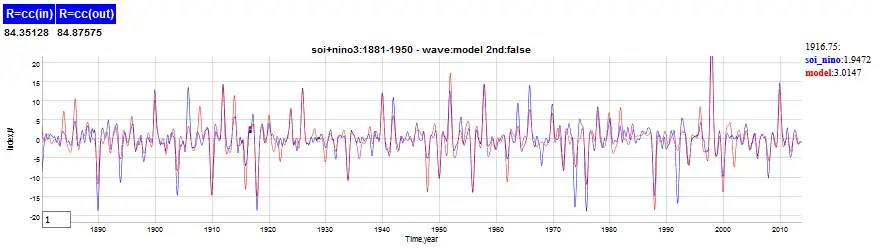Note how well the amplitudes vary in prediction.

In contrast for QBO, a training run from 1953 to 1983 shows this (this may be sign inverted, I don’t care since the positive direction of QBO is defined arbitrarily):The validated model to the right of 1983 shows much less variation with amplitude, in keeping with the excursion-limiting behavior of the sin-of-a-sin formulation (i.e. $$sin(sin(t))$$).

These kinds of approximations are very common in the physics that I was taught, especially in the hairy world of solid-state physics. Simplifying the starting set of equations will not only reduce complexity but it often brings a level of understanding not normally accessible from looking at the original formulation.